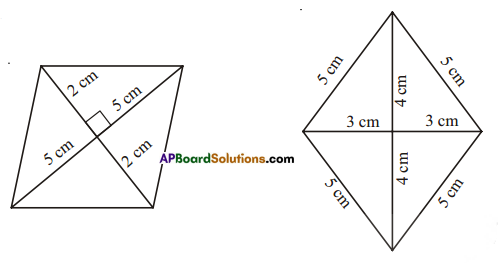# HSSlive: Plus One & Plus Two Notes & Solutions for Kerala State Board

## AP Board Class 7 Maths Chapter 13 Area and Perimeter Ex 4 Textbook Solutions PDF: Download Andhra Pradesh Board STD 7th Maths Chapter 13 Area and Perimeter Ex 4 Book AnswersAP Board Class 7 Maths Chapter 13 Area and Perimeter Ex 4 Textbook Solutions PDF: Download Andhra Pradesh Board STD 7th Maths Chapter 13 Area and Perimeter Ex 4 Book Answers

## Andhra Pradesh State Board Class 7th Maths Chapter 13 Area and Perimeter Ex 4 Books Solutions

 Board AP Board Materials Textbook Solutions/Guide Format DOC/PDF Class 7th Subject Maths Chapters Maths Chapter 13 Area and Perimeter Ex 4 Provider Hsslive

2. Click on the Andhra Pradesh Board Class 7th Maths Chapter 13 Area and Perimeter Ex 4 Answers.
3. Look for your Andhra Pradesh Board STD 7th Maths Chapter 13 Area and Perimeter Ex 4 Textbooks PDF.
4. Now download or read the Andhra Pradesh Board Class 7th Maths Chapter 13 Area and Perimeter Ex 4 Textbook Solutions for PDF Free.

## AP Board Class 7th Maths Chapter 13 Area and Perimeter Ex 4 Textbooks Solutions with Answer PDF Download

Find below the list of all AP Board Class 7th Maths Chapter 13 Area and Perimeter Ex 4 Textbook Solutions for PDF’s for you to download and prepare for the upcoming exams:

Question 1.
Find the area of the following rhombuses.Solution:
Area = 12d1d2
d1 = 5 + 5 = 10cm
d2 = 2 + 2 = 4cm
A = 12 × 10 × 4 = 20 cm2

d1 = 3 + 3 = 6 cm
d2 = 4 + 4 = 8cm
Area = 12d1d2
= 12 × 6 × 8
= 24 cm2

Question 2.
Find the missing values.

 Diagonal – 1 (d1) Diagonal – 2 (d2) Area of the rhombus 12cm 16cm 27mm 2025mm2 14m 57.6m

Solution:

 Diagonal – 1 (d1) Diagonal – 2 (d2) Area of the rhombus 12cm 16cm 12 × 12 × 16 = 96cm2 27mm 2025×227 = 150mm 2025mm2 14m 57.6m 12 × 24 × 57.6 = 691.2 m2

Question 3.
If length of diagonal of a rhombus whose area 216 sq. cm. is 24 cm. when find the length of second diagonal.
Solution:
Given: Length of one diagonal d1 = 24cm, d2 =?
Area = 12d1d2 = 216
12 × d1 × d2 = 216
12 × 24 × d2 = 216
d2 = 21612 = 18

Question 4.
The floor of a building consists of3 000 tiles which are rhombus shaped. The diagonals of each of the tiles are 45 cm and 30 cm. Find the total cost of polishing the floor, if cost per m2 is Rs.2.50.
Solution:
Diagonals of each (shape / rhombus) tiles
d1 = 45cm, d2 = 30cm
Total tiles = 3000
Total area = 3000 × Area of each tile = 3000 × 12 × d1 × d2
= 3000 x 12 × 45 × 30 = 2025000 cm2
= 2025000100×100 m2 = 202.5m2
∴ Cost of polishing the floor at the rate of Rs. 2.50 per a square metre 202.5 × 2.50 = Rs. 506.25

## Andhra Pradesh Board Class 7th Maths Chapter 13 Area and Perimeter Ex 4 Textbooks for Exam Preparations

Andhra Pradesh Board Class 7th Maths Chapter 13 Area and Perimeter Ex 4 Textbook Solutions can be of great help in your Andhra Pradesh Board Class 7th Maths Chapter 13 Area and Perimeter Ex 4 exam preparation. The AP Board STD 7th Maths Chapter 13 Area and Perimeter Ex 4 Textbooks study material, used with the English medium textbooks, can help you complete the entire Class 7th Maths Chapter 13 Area and Perimeter Ex 4 Books State Board syllabus with maximum efficiency.

## FAQs Regarding Andhra Pradesh Board Class 7th Maths Chapter 13 Area and Perimeter Ex 4 Textbook Solutions

#### Can we get a Andhra Pradesh State Board Book PDF for all Classes?

Yes you can get Andhra Pradesh Board Text Book PDF for all classes using the links provided in the above article.

## Important Terms

Andhra Pradesh Board Class 7th Maths Chapter 13 Area and Perimeter Ex 4, AP Board Class 7th Maths Chapter 13 Area and Perimeter Ex 4 Textbooks, Andhra Pradesh State Board Class 7th Maths Chapter 13 Area and Perimeter Ex 4, Andhra Pradesh State Board Class 7th Maths Chapter 13 Area and Perimeter Ex 4 Textbook solutions, AP Board Class 7th Maths Chapter 13 Area and Perimeter Ex 4 Textbooks Solutions, Andhra Pradesh Board STD 7th Maths Chapter 13 Area and Perimeter Ex 4, AP Board STD 7th Maths Chapter 13 Area and Perimeter Ex 4 Textbooks, Andhra Pradesh State Board STD 7th Maths Chapter 13 Area and Perimeter Ex 4, Andhra Pradesh State Board STD 7th Maths Chapter 13 Area and Perimeter Ex 4 Textbook solutions, AP Board STD 7th Maths Chapter 13 Area and Perimeter Ex 4 Textbooks Solutions,
Share: